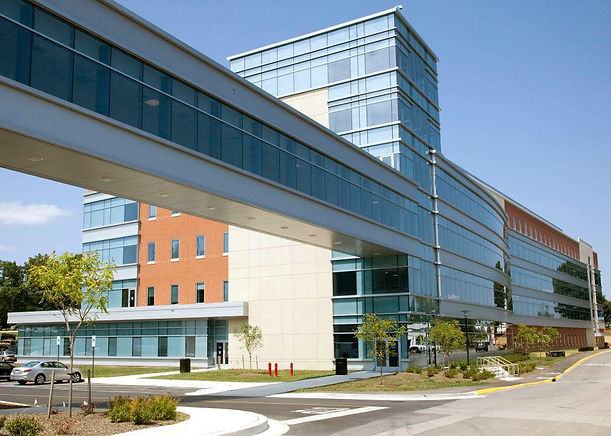## Applied Physics Lab

###### Experiment 1-Fly wheel

PDF of the experiment -

Exp.1

Video Explanation of this Experiment -

###### Experiment 2-Carry Foster

PDF of the experiment -

Exp.2

Video Explanation of this Experiment -

###### Experiment 3-​Modulus of rigidity of the material of wire.

PDF of the experiment -

Exp3

Video Explanation of this Experiment -

###### Experiment 4-To study resistance of a semiconductor and hence to determine the energy gap

PDF of the experiment -

exp4

Video Explanation of this Experiment -

###### Experiment 5(a)-​To study the current versus potential difference characteristics of the light-emitting diode and to determine the cut in voltage

PDF of the experiment -

exp5(a)

Video Explanation of this Experiment -

###### Experiment 5(b)-To study the V-T and pour characteristics of the given solar cell and tho determine its fill factor.

PDF of the experiment -

exp5(b)

Video Explanation of this Experiment -

###### Experiment 6-To determine the coefficient of the thermal conductivity K of rubber in the form of a tube

PDF of the experiment -

exp6

Video Explanation of this Experiment -

###### Experiment 7-To convert the western type galvanometer into an ammeter and voltmeter.

PDF of the experiment -

exp7

Video Explanation of this Experiment -

###### Experiment 8-To draw the graph between the Thermo emf generated and temperature diff. between the hot and cold junction of a copper constantan Thermo capsules

PDF of the experiment -

exp8

Video Explanation of this Experiment -

###### Experiment 9-To find the shape of the platform operating voltmeter of GM counter and the use of G.M counter for the determination of absorption coefficient of copper for the gamma range.

PDF of the experiment -

exp9

Video Explanation of this Experiment -